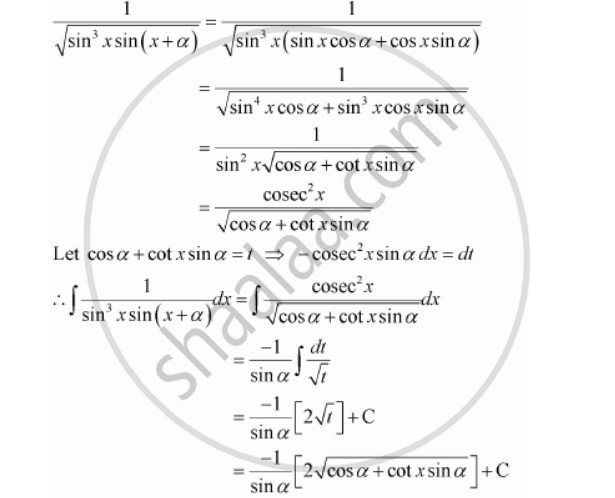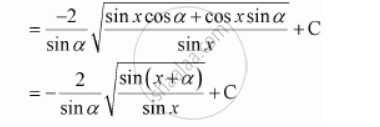Share

# Integrate the Functions 1/Sqrt(Sin^3 X Sin(X + A)) - CBSE (Science) Class 12 - Mathematics

ConceptIntegration as an Inverse Process of Differentiation

#### Question

Integrate the functions 1/sqrt(sin^3 x sin(x + a))

#### SolutionIs there an error in this question or solution?

#### Video TutorialsVIEW ALL 

Solution Integrate the Functions 1/Sqrt(Sin^3 X Sin(X + A)) Concept: Integration as an Inverse Process of Differentiation.
S